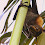## Sunday, September 13, 2015

### An Open(?) Question about Prime Producting Polynomials Part II in 3-D!

(Apologies- No, this post is not in 3-D)

I posted last week about An Open(?) Question about Prime Producing Polynomails

All the math in this post can be found in my writeup  Polynomials and Primes,
unless otherwise specified. NONE of the results are mine.

1) KNOWN: Let  f(x) ∈ Z[x]  be  a poly of degree d. Then there exists a non prime in f(x)'s image (actually there are an infinite number of  non primes, in fact there are an infinite number of composites).  If f(1) is prime then of  f(1+mf(1)) as m=0,1,2,... at most 2d-2 of them are prime.

2) Algorithm to find an x such that f(x) is not prime: compute f(1), f(1+f(1)),...,f(1+(2d-2)f(1)) until you find one that is not prime. This takes 2d-1 evals. OPEN(?): Is there a better deterministic algorithm where we measure complexity by number of evals? Since this is a simple model of computation lower bounds might be possible.

3) There is the following randomized algorithm: Eval f(1)- if its not prime you're done. If f(1) is prime then pick a random m where 0≤ m ≤ (2d-2)2 and eval f(1+mf(1)). This is non prime with prob 1- (1/(2d-1)).

4) What is it about Z that makes this theorem true? In my write up I show that if D is an integral domain with only a finite number of units (numbers  that have mult inverses) then any poly in D[x] has to produce non-primes infinitely often. (A prime in D is a number a such that if a=bc then either b or c is a unit.)

5) What about if D has an infinite number of units? See this  paper for examples of polynomials over integral domains D such that the poly only takes on  only prime or unit values.

6) What about polys over Q? over R? over C? In my write up I prove similar theorems for Q and then use that to get theorems for C.

7)  Looking at polys in Z[x,y] is much harder, see this survey.

8) If f(x)∈Z[x] is a poly then does there exist a prime in f(x)'s image? An infinite number of primes? Easy but stupid answer is no: f(x)=2x. Better question: assume that f(x)'s coefficients are rel prime.

Dirichlet's theorem: if GCD(a,b)=1 then ax+b is prime infinitely  often.

Open: is x2  + 1 prime infinitely often?

#### 1 comment:

1.Dirichlet's theorem is even better! the primes are distributed about equally among the classes (ax + b) parametrized by small a coprime to b.